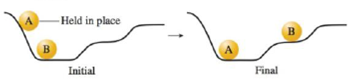# Consider the following diagram when answering the questions below. a. Compare balls A and B in terms of potential energy in both the initial and final setups. b. Ball A has stopped moving in the figure on the right above, but energy must be conserved. What happened to the potential energy of ball A?### Chemistry: An Atoms First Approach

2nd Edition
Steven S. Zumdahl + 1 other
Publisher: Cengage Learning
ISBN: 9781305079243

#### Solutions

Chapter
Section### Chemistry: An Atoms First Approach

2nd Edition
Steven S. Zumdahl + 1 other
Publisher: Cengage Learning
ISBN: 9781305079243
Chapter 7, Problem 27E
Textbook Problem
30 views

## Consider the following diagram when answering the questions below.a. Compare balls A and B in terms of potential energy in both the initial and final setups.b. Ball A has stopped moving in the figure on the right above, but energy must be conserved. What happened to the potential energy of ball A?

(a)

Interpretation Introduction

Interpretation: The potential energy of given diagrams should be explained.

Concept Introduction: potential energy is the energy of an object result of its position. For the gravitational potential energy has,

Formula:

PEgrav = mass × g × height......(1)

### Explanation of Solution

Given:

(b)

Interpretation Introduction

Interpretation: The potential energy of given diagrams should be explained.

Concept Introduction: potential energy is the energy of an object result of its position. For the gravitational potential energy has,

Formula:

PEgrav = mass × g × height......(1)

### Still sussing out bartleby?

Check out a sample textbook solution.

See a sample solution

#### The Solution to Your Study Problems

Bartleby provides explanations to thousands of textbook problems written by our experts, many with advanced degrees!

Get Started

Find more solutions based on key concepts
A properly planned diet controls calories by excluding snacks. T F

Nutrition: Concepts and Controversies - Standalone book (MindTap Course List)

What is motion?

An Introduction to Physical Science

Why do distant stars look redder than their spectral types suggest?

Horizons: Exploring the Universe (MindTap Course List)

Would you need a truck to transport 1025 atoms of copper? Explain.

Introductory Chemistry: An Active Learning Approach

What is a phylum?

Oceanography: An Invitation To Marine Science, Loose-leaf Versin

Two 1.50-V batterieswith their positive terminals in the same directionare inserted in series into a flashlight...

Physics for Scientists and Engineers, Technology Update (No access codes included)